# State assessment for bearing rotor static unbalance based on Welch-PSD and SAE

## Xue Liu1, Jian Ma2, Tong Zhang3

1, 2, 3School of Reliability and Systems Engineering, Beihang University, Beijing, 100191, China

2Corresponding author

Vibroengineering PROCEDIA, Vol. 19, 2018, p. 66-70. https://doi.org/10.21595/vp.2018.20169
Received 17 August 2018; accepted 24 August 2018; published 24 September 2018

33rd International Conference on Vibroengineering in Zittau, Germany, September 24-26th, 2018

Copyright © 2018 Xue Liu, et al. This is an open access article distributed under the Creative Commons Attribution License, which permits unrestricted use, distribution, and reproduction in any medium, provided the original work is properly cited.
Views 69
CrossRef Citations 0
Abstract.

Bearings are an important part of mechanical equipment and it will cause a series of mechanical failures once the malfunction of bearing occurs. Rotor unbalance is the most common type of bearing failure; thus the assessment of bearing rotor unbalance is essential to maintain the normal operation of mechanical. In this paper, a method based on Welch power spectral density estimate (Welch-PSD) and stacked automatic encoder (SAE) is proposed to achieve state assessment of bearing rotor static unbalance by processing the two-way vibration signals collected by the acceleration sensor installed in the vertical and horizontal directions of the bearing. Firstly, the Welch-PSD method is used to decompose the vibration signal to obtain the power spectral density, and the vibration power of the working frequency is taken as the feature. Then, the Stacked Auto-Encoder method is introduced to assessment the bearing rotor unbalance state. This paper designs an experiment of rotor unbalance fault in different degree to verify the accuracy of the designed method. The experimental results show that the Welch-PSD method can accurately extract the rotor unbalance fault feature. In addition, the SAE neural network can apply the fault feature to accurately assessment the bearing rotor unbalance degree.

Keywords: state assessment, static unbalance, bearing rotor, Welch-PSD, stacked automatic encoder.

#### 1. Introduction

As a key component in mechanical equipment, the bearings directly affect the reliability and life of the equipment, thus the bearing has always been an important research object in the field of equipment state monitoring. The rotor is one of the most vulnerable parts as the core component of bearing. Many factors can cause the central inertia spindle of the rotor to deviate from its rotation axis, causing rotor unbalance faults, which become the main vibration excitation source for machinery. Therefore, it is of great significance to assessment the unbalanced state of the bearing rotor to ensure the stable and reliable operation of the equipment.

Researchers around the world have carried out in-depth research on bearing state assessment. Qiu and Lee et al. established a performance degradation assessment method for rolling bearings based on optimal wavelet filter and self-organizing feature mapping . Ben Ali et al. combined EMD with artificial neural network to achieve the classification of rolling bearing defects and performance degradation evaluation of the whole life . Soualhi et al. used the combination of support vector machine and support vector regression as well as the combination of data- Driven and experience-driven methods to achieve the detection of bearing degradation . In terms of the rotor unbalance fault, X. Shen et al. investigated vibration characteristics of a rub-impact rotor-bearing system excited by mass unbalance .

Although many scholars have conducted in-depth research on the intelligent assessment of bearing state and the vibration influence of the bearing rotor unbalance, the state assessment of the bearing under different degrees of rotor unbalance conditions has not been fully realized. In this paper, a bearing rotor unbalance state assessment method based on Welch-PSD and SAE is proposed. The vibration signal is decomposed using the Welch-PSD method to obtain the vibration power at different frequencies, and the vibration power of the working frequency is taken as the signal feature. Then the SAE neural network is used to construct the state assessment mode to assessment the bearing rotor unbalance degree. Finally, this paper designs the bearing rotor unbalance experiment with different failure degrees, and the designed state assessment method is verified by the experiment data. The result shows that the state assessment method proposed in this paper can accurately assessment rotor unbalance faults with different degrees.

#### 2.1. Feature extraction based on Welch-PSD

(1) Welch power spectral density estimate (Welch-PSD).

The Welch-PSD method is a power spectral density estimate method based on the revision of the Periodogram-PSD method and the Bartlett-PSD method. The Welch-PSD method allows the data segments to partially overlap, and the data window of each segment may not be rectangular, to improve the spectral distortion caused by rectangular window. Each segments power spectral density can be expressed as:

(1)
${\stackrel{^}{P}}_{PER}^{i}\left(\omega \right)=\frac{1}{MU}{\left|\sum _{n=0}^{M-1}{x}_{N}^{i}\left(n\right){d}_{2}\left(n\right){e}^{-j\omega n}\right|}^{2},$

where $U=\frac{1}{M}\sum _{n=0}^{M-1}{d}_{2}^{2}\left(n\right)$ is a normalization factor, ensuring that the spectra is asymptotically unbiased estimates. ${d}_{2}\left(n\right)$ is a window function. By averaging the period graph of $L$ segment, we can obtain the power spectrum estimation of the whole signal ${x}_{N}\left(n\right)$:

(2)
${\stackrel{~}{\stackrel{^}{P}}}_{PER}\left(\omega \right)=\frac{1}{L}\sum _{i=1}^{L}{\stackrel{^}{P}}_{PER}^{i}\left(\omega \right)=\frac{1}{MUL}{\left|\sum _{n=0}^{M-1}{x}_{N}^{i}\left(n\right){d}_{2}\left(n\right){e}^{-j\omega n}\right|}^{2}.$

(2) Feature extraction for bearing rotor static unbalance.

In the condition of unbalance fault of bearing rotor, the frequency spectrum of vibration signal is dominated by the bearing working frequency. Due to the nonlinear relationship, some harmonic components are involved. The unbalance vibration feature of the bearing rotor is the power value of the bearing rotating frequency in the vibration signal power spectrum, that is:

(3)
${F}_{E}={\stackrel{~}{\stackrel{^}{P}}}_{PER}\left({\omega }_{W}\right).$

#### 2.2. State assessment based on SAE

An auto-encoder neural network is an unsupervised learning algorithm, which includes an encoder and a decoder. As shown in Fig. 1, the input data is first encoded by ${W}_{1}$, the original $d$-dimensional input $x$ is mapped to the $k$-dimensional hidden layer $h$, and then ${W}_{2}$ is decoded to map $h$ back to the $d$ dimensional vector as close as possible to the original input of the encoder. Auto-encoder is a deep structure when the number of hidden layers of the auto-encoder is greater than 1. The output of the previous layer of the encoder (the middle hidden layer) is used as the input of the subsequent layer. Coding part overlay. That is, stacked automatic encoder.

In this paper, the SAE network is fine-tuned. The bearing rotor unbalance state label is added at the top of the neural network, and the unsupervised features are adjusted by a supervised method. The state assessment network is shown in Fig. 2. After the SAE model is trained, the model is fine-tuned using the unbalance state label to obtain the bearing vibration feature-rotor unbalance state neural network.

Fig. 1. Basic structure of auto-encoder neural network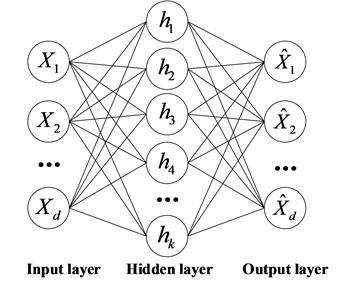Fig. 2. Basic Stacked structure of auto-encoder neural network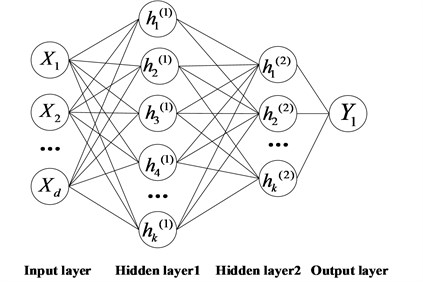#### 3.1. Data description

This paper uses data acquisition from the Machinery Fault Simulator (MFS) test-bed designed by Spectra Quest. In this experiment, 8 kinds of rotor unbalance failure experiments and a normal experiment were designed, and the fault injection position and bearing vibration signal collection position are shown in Fig. 3. Two fault injection holes are distributed on the rotor of the MFS test-bed at intervals of 20° to inject different degrees of unbalanced faults into the bearing rotor. This experiment changed the unbalance of the rotor by changing the angle position between the two counterweight screws. The fault injection position distribution and fault degree are shown in Table 1. The working frequency of the experimental bearing is 30 Hz, and the sampling frequency is 40000 Hz. Each state (8 fault states and 1 normal state) acquires a 4 s vibration signal.

Fig. 3. Machinery fault simulator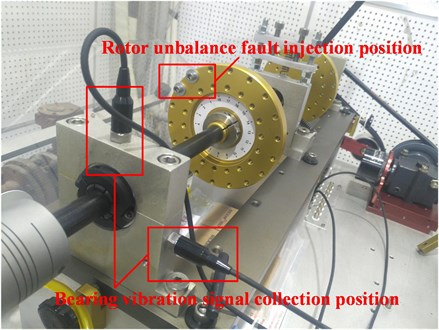#### 3.2. Feature extraction of vibration signals

First of all, the original vibration signal is preprocessed, the noise in the original vibration signal is removed by wavelet packet to obtain bearing vibration information. Then, using the Welch-PSD method, the power spectrum density of the vibration signal can be obtained. The power value corresponding to the working frequency of the bearing is taken as the feature of the bearing vibration signal. The bearing vibration signal feature clustering of 9 states is shown in Fig. 4.

Table 1. Rotor unbalance position and fault degree

 Fault positionUnbalance(g·mm) 0 94.7 183.6 268.5 345.1 Fault position– Unbalance(g·mm) 411.3 465.0 504.5 528.7 –

#### 3.3. State assessment based on SAE

The SAE neural network is trained to obtain the assessment model. The SAE neural network is first trained using normal state feature data and maximum fault degree state feature data. The health of normal working state is defined as 1. The maximum degree state of rotor unbalance is defined as the failure state, and the health is 0. The health is used as the training data label to fine tune the SAE neural network to obtain the rotor unbalance state assessment model. The SAE neural network training parameters are shown in Table 2.

Table 2. SAE neural network training parameters

 Parameter name Learning rate Weight penalty Input layer neurons Hidden layer 1 neurons Hidden layer 2 neurons Output layer neurons Parameter value 0.001 0.3 2 80 80 1

The assessment model is used to assess the test data. The bearing vibration feature data are spliced from low to high according to rotor unbalance as the test data, which is composed to test the accuracy of the assessment model. The assessment results of the rotor unbalance using the assessment model for the test data are shown in Fig. 5. The experimental results show that the SAE neural network can accurately assess rotor unbalance faults in different degrees.

Fig. 4. Clustering result of the fault features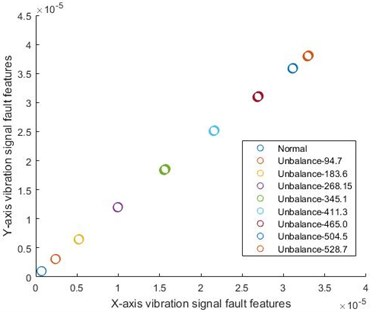Fig. 5. Assessment result of the testing data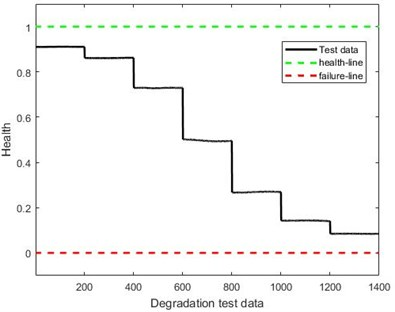#### 4. Conclusions

Bearing plays an important role in mechanical equipment, and bearing rotor unbalance fault is a common fault type of bearing. Therefore, bearing rotor unbalance state assessment research is of great significance. This paper proposes a data-driven method to assess bearing rotor unbalance degree. The designed method has obtained accurate results for the assessment of vibration data of rotor bearings in different degrees. Future work will focus on the evaluation of other faults in bearings.

#### Acknowledgements

This research was supported by the National Natural Science Foundation of China (Grant Nos. 51605014, 51105019 and 51575021), the Aviation Science Fund (Grant No. 20163351018), the Technology Foundation Program of National Defense (Grant No. Z132013B002), and the Fundamental Research Funds for the Central Universities (Grant No. YWF-18-BJ-Y-159).

1. Qiu H., Lee J., Lin J., et al. Robust performance degradation assessment methods for enhanced rolling element bearing prognostics. Advanced Engineering Informatics, Vol. 17, Issues 3-4, 2003, p. 127-140. [Publisher]
2. Ben Ali J., Fnaiech N., Saidi L., et al. Application of empirical mode decomposition and artiﬁcial neural network for automatic bearing fault diagnosis based on vibration signals. Applied Acoustics, Vol. 89, 2015, p. 16-27. [Publisher]
3. Soualhi A., Medjaher K., Zerhouni N. Bearing health monitoring based on Hilbert-Huang transform, support vector machine and regression. IEEE Transactions on Instrumentation and Measurement, Vol. 64, Issue 1, 2015, p. 52-62. [Publisher]
4. Shen X., Jia J., Zhao M. Numerical analysis of a rub-impact rotor-bearing system with mass unbalance. Journal of Vibration and Control, Vol. 13, Issue 12, 2007, p. 1819-1834. [Publisher]